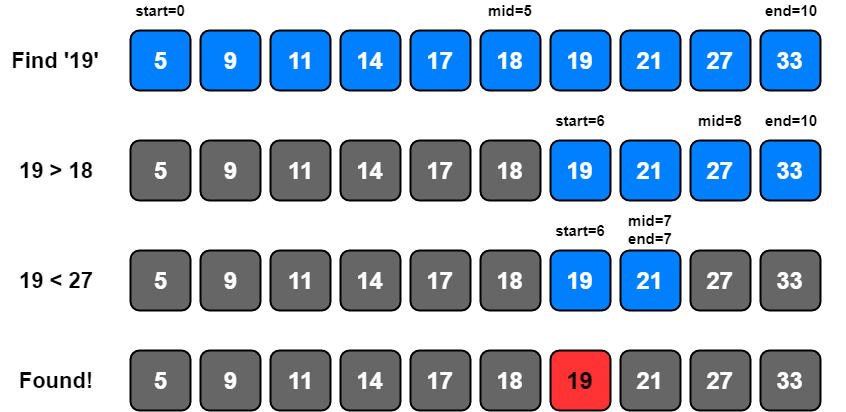# Coding Patterns: Modified Binary Search

In Coding Patterns series, we will try to recognize common patterns underlying behind each algorithm question, using real examples from Leetcode.

Previous posts were about Sliding Window, Two Pointers, Fast & Slow Pointers, Merge Intervals, Cyclic Sort, In-place Reversal of a Linked List, Breadth First Search (BFS), Depth First Search (DFS), Two Heaps and Subsets patterns and today, we will introduce Modified Binary Search pattern which is very useful to solve the problems whenever we are given a sorted Array or Linked List or Matrix, and we are asked to find a certain element.

This pattern describes an efficient way to handle all problems involving Binary Search.

LeetCode 704 - Binary Search [easy]

Given a sorted (in ascending order) integer array `nums` of n elements and a `target` value, write a function to search `target` in `nums`. If `target` exists, then return its index, otherwise return -1.

Example 1:

``````Input: nums = [-1, 0, 3, 5, 9, 12], target = 9
Output: 4
Explanation: 9 exists in nums and its index is 4
``````

Example 2:

``````Input: nums = [-1, 0, 3, 5, 9, 12], target = 2
Output: -1
Explanation: 2 does not exist in nums so return -1
``````

Note:

• You may assume that all elements in `nums` are unique.
• n will be in the `range [1, 10000]`.
• The value of each element in `nums` will be in the `range [-9999, 9999]`.

### Binary Search Solution

1- Let’s assume that `start` is the first element and `end` is the last element in `nums`.

``````start = 0
end = len(nums) - 1
``````

2- We need to find middle value, `mid`. An easy way to do it in Python is

``````mid = (start + end) // 2
``````

For Java and C++, this equation will work for most cases, but when `start` or `end` is large, this equation will give us the wrong result due to integer overflow. To solve this problem, we will do our calculation a bit differently;

``````int mid = start + (end - start) / 2;
``````

3- Next, we will see if the `target` value is equal to the number at `mid` value. If it is equal we return `mid` as the required index.

4- If `target` is not equal to number at index `mid`, there are two possibilities that we need to check:

• If `target < nums[mid]`, then we can conclude that `target` will be smaller than all the numbers after index `mid` as the array is sorted in the ascending order. We can reduce our search to `end = mid - 1`.

• If `target > nums[mid]`, then we can conclude that `target` will be greater than all numbers before index `mid` as the array is sorted in the ascending order. We can reduce our search to `start = mid + 1`.``````from typing import List

class Solution:
def search(self, nums: List[int], target: int) -> int:
start, end = 0, len(nums) - 1
while start <= end:
mid = (start + end) // 2

if target == nums[mid]:
return mid

if target < nums[mid]:
end = mid - 1
else:
start = mid + 1
return -1
``````

Time Complexity: O(log N) where N is the total elements in the given array.

Space Complexity: O(1)

## How to identify?

This approach is quite useful to solve the problems whenever we are given a sorted Array or Linked List or Matrix, and we are asked to find a certain element.

This pattern describes an efficient way to handle all problems involving Binary Search.

Tags:

Categories:

Updated: